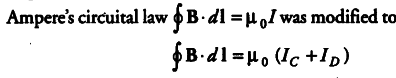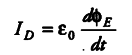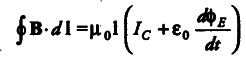# Considering the case of a parallel plate

Considering the case of a parallel plate capacitor being charged, show how one is required to generalise Ampere’s circuital law to include the term due to displacement current.

Ampere’s circuital law, during charging of a capacitor was found inconsistent. Therefore, Maxwell modified Ampere’s circuital law by introducing displacement current.It is called modified Ampere’s circuital law or Ampere-Maxwell’s circuital law. The displacement current arising due to time varying electric field is given byTherefore, modified Ampere’s circuital law may be expressed asThe following inferences can be drawn from the above discussion:
(i) The conduction and displacement currents are individually discontinuous, but the currents together possess the property of continuity through any closed electric circuit.
(ii) The displacement current is precisely equal to the
conduction current when the two present in different parts of the circuit.
(iii) The displacement current arises due to the rate of change of electric flux (or electric field) between the two plates of the capacitor.
(iv) Just as the conduction current, the displacement current is also the source of magnetic field.